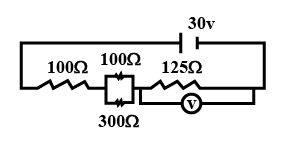# The reading of the voltmeter in this circuit isA. 12.5 V

Explanation

Correct option is A)
Simplified circuit is as drawn below.

$$R_{\text {eq }}=300$$

$$\mathrm{I}=\frac{\mathrm{V}}{\mathrm{R}_{\text {eq }}}=\frac{30}{300}=0.1 \mathrm{~A}$$

$$\text { Reading of voltmeter } =\mathrm{I} \times \mathrm{R}=0.1 \times 125 \\=12.5 \mathrm{~V}$$